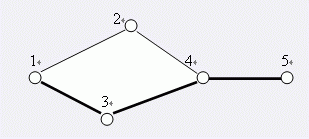# 洛谷 P2542 [AHOI2005]航线规划 题解【树链剖分】【线段树】【tarjan】

一道特别特别特别考基本功的题。

## 题目描述A、B两星球之间，如果某条航线不存在，就无法从A星球抵达B星球，我们则称这条航线为关键航线。

5 5
1 2
1 3
3 4
4 5
4 2
1 1 5
0 4 2
1 5 1
-1

1
3

## 题解：

关键路径让人联想到割边，不过这里求的是两点之间的关键路径，可以缩环为点，缩图为树，求树上两点间距离。而有的环还会被拆开，根据航线被破坏后不能再被修复，我们考虑倒着做。

最终状态的环是可以缩掉的，因为环内各点一定不存在关键路径。这时图变成了一棵树，我们如果再给树上两点连上一条边，就会成环，这样又要做一遍tarjan缩环。不过我们可以用树剖把路径上的点边权改为0，因为上面建模时把缩点后两点间距离定为了1。只要把最短路径上的边全部改为0，这一段就相当于一个环，后面以此类推也可以做。缩环后的处理一定要特别注意，一定要映射为缩点后的点编号。

不过树剖维护的是点权，这里要维护边权，那么就让每个点去维护自己的入边边权。不过要注意的是，树剖两点间LCA的值是不能改的，因为它维护的边权不在最短路径上。这样一来就要特判LCA的位置，如果恰好在同一环上，那就不操作；到树剖最后一步相同链时，区间是[dfn[x]+1,dfn[y]]，因为dfn[x]维护的边不在路径上。注意建树时1点维护的边权为0。

Tip：namespace完美解决了我多个dfn/s数组和cnt的麻烦。

## Code：

#include
#include
#include
#include
#define ls (k<<1)
#define rs (k<<1|1)
#define mid (l+r>>1)
#define Mid (T[k].l+T[k].r>>1)
using std::map;
using std::min;
using std::max;
map >m;
struct edge
{
int n,nxt;
int able;
edge(int n,int nxt)
{
this->n=n;
this->nxt=nxt;
able=1;
}
edge()
{
nxt=-1;
able=1;
}
}e;
{
}
int n;
struct query
{
int u,v,op,ans;
}q;
int del;
namespace Tarjan
{
int dfn,low,cnt=0;
int in,s,top=0;
void tarjan(int x,int from)
{
dfn[x]=++cnt;
low[x]=dfn[x];
in[x]=1;
s[++top]=x;
{
if(e[i].n==from||!e[i].able)
continue;
if(dfn[e[i].n])
{
if(in[e[i].n])
low[x]=min(dfn[e[i].n],low[x]);
else
continue;
}
else
{
tarjan(e[i].n,x);
low[x]=min(low[x],low[e[i].n]);
}
}
if(low[x]==dfn[x])
{
while(s[top]!=x)
{
in[s[top]]=0;
del[s[top--]]=x;
}
in[x]=0;
del[s[top--]]=x;
}
return;
}
}
namespace Cut
{
struct node
{
int l,r,v,lazy;
node(int l,int r,int v)
{
this->l=l;
this->r=r;
this->v=v;
lazy=0;
}
node(){lazy=0;}
}T;
void build(int k,int l,int r)
{
T[k]=node(l,r,r-l+1);
if(l==1)
T[k].v--;
if(l==r)
return;
build(ls,l,mid);
build(rs,mid+1,r);
}
void pushdown(int k)
{
if(T[k].lazy==0||T[k].l==T[k].r)
{
T[k].lazy=0;
return;
}
T[ls].v=0;
T[ls].lazy=1;
T[rs].v=0;
T[rs].lazy=1;
T[k].lazy=0;
return;
}
void change(int k,int l,int r)
{
if(T[k].l==l&&T[k].r==r)
{
T[k].lazy=1;
T[k].v=0;
return;
}
pushdown(k);
if(r<=Mid)
change(ls,l,r);
else if(l>Mid)
change(rs,l,r);
else
{
change(ls,l,Mid);
change(rs,Mid+1,r);
}
T[k].v=T[ls].v+T[rs].v;
return;
}
{
if(l==T[k].l&&r==T[k].r)
return T[k].v;
pushdown(k);
if(r<=Mid)
else if(l>Mid)
else
}
int dfn,top,fa,sz,s,d,cnt=0;
void dfs1(int x)
{
sz[x]=1;
if(!d[e[i].n]&&e[i].able)
{
if(e[i].n==1)
{
int o;
o=1;
}
fa[e[i].n]=x;
d[e[i].n]=d[x]+1;
dfs1(e[i].n);
sz[x]+=sz[e[i].n];
if(sz[e[i].n]>sz[s[x]])
s[x]=e[i].n;
}
}
void dfs2(int x,int t)
{
dfn[x]=++cnt;
top[x]=t;
if(s[x])
dfs2(s[x],t);
if(e[i].able&&e[i].n!=fa[x]&&e[i].n!=s[x])
dfs2(e[i].n,e[i].n);
}
void cut()
{
d=1;
dfs1(1);
cnt=1;
top=1;
if(e[i].able)
dfs2(e[i].n,e[i].n);
}
int modify(int op,int x,int y)
{
int ans=0;
int tx=top[x],ty=top[y];
while(tx!=ty)
{
if(d[tx]dfn[y])
{
int t=x;
x=y;
y=t;
}
if(op==0)
change(1,dfn[x]+1,dfn[y]);
else
return ans;
}
}
int main()
{
int M,u,v;
scanf("%d%d",&n,&M);
for(int i=1;i<=M;i++)
{
scanf("%d%d",&u,&v);
m[u][v]=ecnt-1;
m[v][u]=ecnt;
}
int T;
for(T=1;;T++)
{
scanf("%d",&q[T].op);
if(q[T].op==-1)
break;
scanf("%d%d",&q[T].u,&q[T].v);
if(q[T].op==0)
{
int tmp=m[q[T].u][q[T].v];
e[tmp].able=0;
e[tmp^1].able=0;
}
}
T--;
Tarjan::tarjan(1,1);
for(int i=1;i<=n;i++)
{
e[j].n=del[e[j].n];
if(del[i]==e[j].n||e[j].able==0)
{
e[j].able=0;
continue;
}
if(del[i]!=i)
{
e[j].able=0;
}
}
Cut::cut();
Cut::build(1,1,Cut::cnt);
for(int i=T;i;--i)
{
if(q[i].op==0)
Cut::modify(0,del[q[i].u],del[q[i].v]);
else
q[i].ans=Cut::modify(1,del[q[i].u],del[q[i].v]);
}
for(int i=1;i<=T;i++)
if(q[i].op==1)
printf("%d\n",q[i].ans);
return 0;
}


### 说点什么Subscribe

/* */